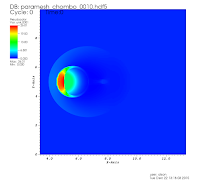Multidimensional Improvements to the NAG Riemann Solvers

Posted on
4 May 2016

The NAG Library contains routines for solving the partial differential equations specific to compressible, ideal fluid flow. These equations are generally written in conservation law form where the conserved quantities are mass density, momentum and total energy of the fluid.  This set of equations can be solved using a finite volume technique that considers each conserved variable as a volume average over a finite volume (typically a small cube) and sums the fluxes (flow rates per unit area) computed at the faces surrounding the volume to get the total rate of change of a particular variable for that volume.

Several methods exist to solve this set of coupled equations (e.g. Flux Corrected Transport, ENO and WENO schemes, etc.). I focus here on the Godunov method where, in its simplest form, the fluxes are computed by solving a Riemann problem at the interface between two cells in the computational mesh. In such methods, appropriately limited (I do not address limiting procedures here, but see e.g. [Toro, 1999]) states left and right of a cell interface are computed. These states are assumed to be constant, discontinuous states, i.e. a Riemann problem. The Riemann problem does have an exact solution which can be used to finally compute the fluxes at the cell interface. The NAG Library possesses a routine for computing the exact solution and returning the fluxes to the user in one spatial dimension (D03PX (link is external)). The NAG Library also possesses three other approximate "Riemann Solvers": Roe’s solver (D03PU (link is external)), Osher’s solver (D03PV (link is external)), and the HLL solver (D03PW (link is external)). Descriptions of all these algorithms can be found in [Toro, 1999]. To make these routines more generally useful they should be able to handle the full set of three dimensional equations and this blog discusses the modifications to these routines to accomplish this.

TESTS

To test my implementations of these Riemann solvers, I have set up and run a test problem. The problem is run in two space dimensions and the domain of the problem is 0 to 40 in the x direction and 0 to 10 in the y direction. The entire domain is initially filled with fluid of density ρ = 1. and given a supersonic velocity of Mach 2.7 in the x direction. Into this flow a circular dense region (a blob) of ρ = 10 and radius 1 is inserted. The boundary conditions are set to produce a constant inflow of Mach 2.7 at the x = 0 boundary and outflow at the x = 40 boundary. Reflecting boundary conditions are set at the y = 0 and y = 10 boundaries. As the simulation proceeds a bow shock forms at the front of the dense blob. The dense blob is compressed and flattened and at later times Raleigh-Taylor and Kelvin-Helmholtz instabilities form at the interface between the dense blob and the supersonic flow. In the figure below, each panel shows a different time in the evolution of the mass density of the blob as it interacts with the supersonic flow surrounding it.

Results using the modified NAG HLL solver are shown in the next set of figures. The results are virtually identical to those from the original code. The rest of the NAG Riemann solvers that I have modified give similar results.REFERENCES

P. MacNeice, K. M. Olson, C. Mobarry, R. de Fainchtein, and C. Packer. Paramesh: A parallel adaptive mesh refinement community toolkit. NASA Tech. Rep. CR-1999- 209483, 1999.

P. MacNeice, K. M. Olson, C. Mobarry, R. de Fainchtein, and C. Packer. Paramesh: A parallel adaptive mesh refinement community toolkit. Comput. Phys. Commun., 126: 330–354, 2000.

K. Olson. Paramesh: A parallel adaptive grid tool. In A. Deane, A. Ecer, G. Brenner, D. Emerson, J. McDonough, J. Periaux, N. Stofuka, and D. Tromeaur-Dervout, editors, ”Parallel Computational Fluid Dynamics, Theory and Applications”. Elsevier, 2006.

K. Olson and P. MacNeice. An overview of the paramesh amr software package and some of its applications. In T. Plewa, T. Linde, and G. V. Weirs, editors, ”Adaptive Mesh Refinement Theory and Applications, Proceedings of the Chicago Workshop on Adaptive Mesh Refinement Methods”, volume 41 of Lecture Notes in Computational Science and Engineering, pages 315–330. Springer, 2005.

E. F. Toro. Riemann Solver and Numerical Methods for Fluid Dynamics, A Practical Introduction. Springer, Berlin, 1999.

B. van Leer, A New Approach to Numerical Gas Dynamics, "Computing in Plasma Physics and Astrophysics", Max-Planck-Institute fur Physik, Garching, Germany, April 1976.